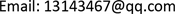1成都信息工程大学，大气科学学院，高原大气与环境四川省重点实验室，四川 成都

2贵州省气象台，贵州 贵阳1. 引言

2. 数据与研究区域概况2.1. 数据来源

2.2. 研究区域概况

Area statistics of a type of functional are

3. 研究方法3.1. 大气稳定度计算

h 0 = arcsin [ sin ∅ sin σ + cos ∅ cos σ cos w ] (1)

w = 15 × ( S T − 12 ) (2)

ST为真太阳时，公式如下：

S T = hour + min 60 + lon − 120 15 (3)

h 0 = arcsin [ sin ∅ sin σ + cos ∅ cos σ cos ( 15 t + λ − 300 ) ] (4)

σ = [ 0.006918 − 0.39912 cos θ 0 + 0.70257 sin θ 0 − 0.006758 cos θ 0     + 0.000907 sin 2 θ 0 − 0.002697 cos 3 θ 0 + 0.001480 sin 3 θ 0 ] × 180 π (5)

≤4/≤4−2−1+1+2+3
5~7/≤4−10+1+2+3
≥8/≤4−100+1+1
≥5/5~70000+1
≥8/≥800000

Atmospheric stability leve

+3+2+10−1−2
≤1.9AA~BBDEF
2~2.9A~BBCDEF
3~4.9BB~CCDDE
5~5.9CC~DDDDD
≥6DDDDDD

3.2. 混合层厚度计算

L = a 0 u 10 f (6)

L = b 0 × u 10 f (7)

3.3. A值法

A值法更适用于地区级范围的容量计算，其原理是通过算出控制区的总面积和其中各功能区的面积，以及当地的基本限值系数，就能估算出该研究区允许排放的总量。A值法是以地面大气环境质量为目标值，使用简便的箱模式而实现的具有宏观意义的总量控制。A值的计算将通风量和天数结合在一起，简化输送扩散项的计算。

3.4. 季节大气环境容量核算

C = q a Δ x u H F (8)

c ¯ = u ¯ c b + Δ x q a H i u ¯ + ( u d + w r R + H i T c ) Δ x H i (9)

q s = c s ( u ¯ H i Δ x + u d + w r R ) (10)

Δ x = 2 S π (11)

Q a = q s ⋅ S ⋅ T (12)

Q a = 3.1536 × 10 − 3 c s π v E S 2 + 3.1536 × c s S ( u d + u w ) + c s S × 0.639 × H i T 1 2 (13)

∂ = t 365 × 3.1536 (14)

Seasonal coefficient values of the study are

3.5. 大气环境容量核算

h ¯ = n ∑ i = 1 n 1 h i (15)

u 2 = u 1 ( z 2 z 1 ) p , z 2 ≤ 200   m ; u 2 = u 1 ( 200 z 1 ) p , z 2 > 200   m (16)

u = 1 H i ∫ 0 H i u ( z ) d z = 1 H i [ ∫ 0 200 u 10 ( z 10 ) p d z + ∫ 200 H i u 10 ( 200 10 ) p d z ] (17)

p为地表摩擦系数，贵阳市属城市取系数0.4。

v E = 3 1 u i − 1 H i − 1 + 1 u i H i + 1 u i + 1 H i + 1 (18)

Ventilation volume in each season of the study are

A = a i × 10 − 3 π v E 2 , i = 1 , 2 , 3 , 4 (19)

Statistical A value of each element of the study area (104 km2

A值0.7472050.6103380.6416610.588639

4. 贵阳市季节大气环境容量4.1. 季节基本大气环境容量(静态及输送扩散清除项)

Q a 1 = A ∑ i = 1 n c s b S i S (20)

Q a 1 = A × [ 0.8 × c s 1 × S 1 S + ( c s 2 − 0.5 × c s 1 ) × S 2 S ] (21)

Basic environmental capacity of different seasons in the study area (104 t∙a−1

SO23.2943572.6909212.8290212.595255
NO21.3586581.1097891.1667441.070334
PM10 3.3199292.7118082.850982.6154
PM2.5 1.8170111.4841841.5603531.431419

4.2. 季节干沉降项

Q a 2 = a i × c s S i u d (22)

Q a 2 = a i × ( c s 1 S 1 + c s 2 S 2 ) u d (23)

Dry deposition of pollutants in Guiyang city in different seasons (104 t∙a−1

SO21.3197391.3197391.3053941.2910495.23592
NO0.178810.178810.1768660.1749230.709409
PM101.9467921.9467921.9256311.9044717.723687
PM2.50.5511610.5511610.545170.5391792.186672

4.3. 季节湿沉降项

Q a 3 = a i × c s S i u w (24)

Q a 3 = a i × ( c s 1 S 1 + c s 2 S 2 ) × w r × R (25)

Q a 3 = 3.1536 × C S × S i × K w (26)

Dry deposition of pollutants in Guiyang city in different seasons (104 t∙a−1

SO21.3197391.3197391.3053941.2910495.23592
NO0.178810.178810.1768660.1749230.709409
PM101.9467921.9467921.9256311.9044717.723687
PM2.50.5511610.5511610.545170.5391792.186672

4.4. 季节化学清除项

Q a 4 = a i × c s S i × 0.639 × H i T 1 2 (27)

Chemical removal items of pollutants in Guiyang City in different seasons (104 t∙a−1

SO21.5492861.3854431.3441681.2138995.492796
NO20.1457720.1303560.1264720.1142150.516814

4.5. 年环境容量汇总及对比

Total atmospheric environmental capacity of each season in the study area (104 t∙a−1

SO23.2943571.31973911.377971.54928617.54135100.0992
2.6909211.31973953.972361.38544359.36846
2.8290211.30539411.765981.34416817.24456
2.5952551.2910490.8446721.2138995.944875
NO21.3586580.178811.9269840.1457723.61022319.1353
1.1097890.178819.1408150.13035610.55977
1.1667440.1768661.9926980.1264723.46278
1.0703340.1749230.1430550.1142151.502527
PM103.3199291.9467929.538086-14.8048154.92909
2.7118081.94679215.725620.3842
2.850981.9256328.73496313.51157
2.61541.9044711.7086376.228508
PM2.51.8170110.5511614.752617-7.12078926.27179
1.4841840.5511617.8357199.871064
1.5603530.545174.3524396.457962
1.4314190.5391790.8513762.821975

Average atmospheric environmental capacity of Guiyang area (104 t∙a−1

5. 结论与讨论

1) 贵阳市春夏秋冬的A值分别为0.747205、0.610338、0.641661和0.588639。由混合层高度的日变化可知，其峰值多出现在下午。对于季节性而言，混合层平均厚度在春季达到最大值。

2) 四项污染因子的基本环境容量变化趋势较为相近。其中，春季容量最大，冬季最小。对于四项污染因子的干沉降项而言，干沉降项季节性差异较小。湿沉降项是造成各污染物总容量差异的主要原因，SO2在夏季有着极高的湿沉降值。作为湿沉降的主导因子，降雨量在夏季最大，对应湿沉降值也较大，冬季则因少雨较小。

3) 贵阳市的SO2、NO2、PM10和PM2.5的年总大气环境容量分别为100.1、19.1、55.0和26.3 (104 t∙a−1)。四项污染因子中SO2的大气环境容量最大，NO2的最小。四项清除项中，湿沉降是最主要的清除方式，而干沉降项和化学清除项最小。

4) 造成贵阳市各个季节大气环境容量差异的主要因素为各季节通风量和湿沉降的时间分布不均匀。而由于降雨量、通风量和一二类功能区所占比例的不同，使得贵阳的大气环境容量较同属西南地区的成都略大。